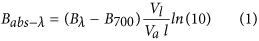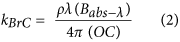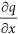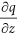## Introduction

Kanpur and its surrounding regions are known for high aerosol loadings mainly sourced by open biomass and trash burning that cause large-scale particulate matter accumulation, especially during winter1. Mass concentrations of PM2.5 (particulate matter less than 2.5 μm in diameter) were 187 ± 90 μg m−3 (mean ± standard deviation), which is 7 times higher than World Health Organization (WHO) standards2. This study reports submicron (PM1) carbonaceous aerosol light absorption properties using both offline and online measurements. Black carbon (BC) and brown carbon (BrC) are the two major absorbing carbonaceous aerosols in the atmosphere. BC and BrC are mainly distinguished by their optical (absorption, scattering etc.) and physical (volatility, color etc.) properties3. The ratio of BC to organic mass determine the color of the particles which is in turn influenced by the burning conditions4. BC is dark in color and shows strong light absorbing capacity throughout the spectra. However, BrC is brown in color and its absorption is limited to UV and lower visible wavelengths5. Aerosol absorption depends on the mass, mixing state, chemical composition and the refractive index of the species present in the atmosphere. The refractive index indicates an aerosol’s capacity to scatter and absorb light and is usually expressed in a complex form as m = n + ik, where the real term ‘n’ indicates scattering and the imaginary term ‘k’ indicates absorption. The mixing state is mainly classified as external or internal, with ‘external mixing’ describing the coexistence of physically separated particles and ‘internal mixing’ describing a state of homogeneous mixing that may also include the formation of core-shell structures6. In core-shell type structures, the shell acts like a lens and focuses light towards the core, causing an enhancement in absorption (lensing effect)7. The magnitude of enhancement caused by this lensing effect depends on the thickness and chemical properties of the materials that form the shell.

Due to its refractory nature and specific source-sink profile BC refractive index is well characterized and fairly constant6. Unlike BC, BrC sources vary from direct emissions, mostly from biomass burning, to secondary formation in the atmosphere. BrC is also influenced by photochemical aging, which leads to substantial spatial and temporal variability downwind of sources8. The higher concentration of BrC combined with internal mixing between BC and inorganics results in increased absorption1,9. Optical properties of BrC also depend on the source, burning temperature and local meteorological conditions10. Due to above factors contribution from BrC to total aerosol absorption might vary significantly. A previous aerosol absorption measurement study in Kanpur reports a roughly 9-fold increase in total absorption at 405 nm during a biomass burning event compared to clear periods11. The direct radiative forcing was found to increase by ~35% during biomass burning, in which BrC alone contributed up to 20%11. Additionally, global climate model inputs are lacking key parameters such as BrC refractive indices (kBrC) and estimates of core-shell coating thickness, which are often used to estimate the overall aerosol mass absorption efficiency in radiative forcing estimates.

## Results and Discussion

### BrC Refractive Index

PM1 was collected on quartz filters (no. of samples = 57) for 8-hour sampling durations (3 filters/day) during the winter season (23-Dec-2014 to 24-Feb-2015) and were then analyzed for kBrC values. Reported kBrC values are elevated compared to previous measurements (Fig. 1)12. Reference 12 reported a kBrC value of 0.0082 (350 nm); our study observed a kBrC 5 times higher at 0.042 for a slightly longer wavelength (365 nm). At 405 nm, ref. 8 reported a kBrC value of 0.009 during a biomass burning event in Colorado, USA; the average kBrC value from our study was 4 times higher at 0.037. A number of previous studies reported kBrC values higher than this study13,14,15. However, these studies are done on lab generated aerosols from a single source with higher absorbing capacity. This study measured atmospheric BrC, originated from various emission sources and secondary processes. High kBrC values suggest that atmospheric BrC might be substantially more absorbing over urban India than other previously measured locations in the United States.

### Enhancement in Absorption

Using an online instrument (PASS 3) ambient air volumes were directly assessed for total absorption coefficients in Mm−1 at 405 and 781 nm before and after passing through a thermal denuder (Supplementary Fig. S1). Denuded aerosols were heated to 300 °C presumably leaving BC as the only remaining species contributing to absorption (potential residual coating effects are discussed later)16. The ratio between atmospheric and denuded absorption, defined as the absorption enhancement (Eabs), provides an estimate of the contribution from lensing effects and externally-mixed BrC to total absorption. Average Eabs values at 405 and 781 nm are 1.21 ± 0.22 and 1.27 ± 0.14, respectively. Both lensing and externally-mixed BrC contribute to Eabs at 405 nm. At 781 nm lensing is the only contributing species as expected since BrC absorption is negligible at longer wavelengths17. Eabs_405 shows a strong diurnal variation while Eabs_781 demonstrates minimal variation (Fig. 2). Daytime Eabs_405 is lower than the corresponding Eabs_781, which is similar to reported trends observed by a recent study at 870 nm18. Also, ref.18 reports diurnal variations with lower daytime kBrC at 405 nm. These lower daytime Eabs_405 values have been attributed to the combined effects of low kBrC and possible photobleaching of BrC19,20. These low kBrC values are due to less primary emissions and non-absorbing secondary organic aerosols formed via photochemical reactions during the daytime.

Photochemical reactions during daytime are known to convert primary organics to semi-volatile oxygenated organics (SV-OOA) and then further oxidize them to low volatile oxygenated organics (LV-OOA)21. Analysis of high-resolution organic aerosol mass spectra from an Aerosol Mass Spectrometer (AMS) provides atomic oxygen to carbon (O:C) and hydrogen to carbon (H:C) ratios, which provides information about the degree of oxygenation. Photochemical reactions that occur with organic particulates in the atmosphere transform the compounds with low O:C values (hydrocarbon-like aerosols, HOA) to high O:C compounds (LV-OOA). Figure 3 shows a Van Krevelen diagram22 of color-coded Eabs_405 values at various O:C and H:C ratios; diurnal variations of O:C and H:C are also plotted. H:C ratios are lowest during daytime hours when O:C ratios are elevated. Higher Eabs_405 values are associated with lower O:C and higher H:C values indicating semi to moderately volatile aerosols. LV-OOA compounds with high O:C values are contributing less to Eabs_405. This result differs from a lab study10 that reported LV-OOA to be highly absorbing in nature. In this study, Eabs_405 was high during nighttime hours when direct emissions did not experience photochemical aging, thereby maintaining high H:C and low O:C ratios.

To further understand the contribution of different species to total absorption, we used Mie theory to calculate optical properties using measured parameters as inputs, keeping a core-shell particle structure. In core-shell modeling, BC is assumed to form the spherical core and a mixture of organic and inorganic species as a shell. Assuming spherical BC with perfect shell in modeling might not reflect the actual atmospheric conditions. Freshly emitted BC is fractal in shape and its optical properties are different when compared to a spherical BC23. However, the initial fractal shape of BC is known to collapse and form a spherical shape within a time frame of 4 to 6 hours24. The study area (IIT Kanpur campus) has no nearby BC sources and winter season in Kanpur is known for high aerosol loading and relative humidity which favors mixing of aerosols, supporting the formation of spherical BC1. Therefore, it is safe to assume that the BC present in the sampling site is spherical in shape. We have also considered the externally mixed BC and BrC, which will also contribute the total absorption. For core-shell particles, determining the thickness of the coating is important to understand the subsequent optical properties. This coating thickness is represented by the “coating factor” (CF), which is the ratio of coated particle diameter to core-only (i.e., no shell) particle diameter. Higher CF values indicate thickly coated particles, which results in higher absorption through lensing.

A number of studies have reported CF values using Mie modeling8,18,25 using assumptions that the entire BC size distribution is internally mixed and uniformly coated (Mie Model CF-Case 1). In reality, some fraction of BC is always externally mixed and coating should therefore only be applied to remaining internally-mixed fraction. We divided the total BC number size distribution into external and internal mixtures. We employed the signal analysis technique to calculate the time lag between scattering and incandescence signal from SP226, which provides the percentage of coated BC particles (see ref. 26 for details). Results show that during daytime 75% of BC is coated compared to 55% for nighttime. This diurnal change in the BC mixing state is considered by the CF calculation in this study (Mie Model CF-Case 2). Mean CF values from Mie Model CF-Case 1 and 2 are 1.34 ± 0.15 and 1.60 ± 0.31, respectively. Since Mie Model CF-Case 1 assumes that all BC is uniformly coated (rather than just the internally-mixed fraction), the resultant CF is less than Mie Model CF-Case 2. Case 1 CF values are, on average, 16% lower than case 2 CF values; this difference attributes a 7% contribution to absorption from lensing effects. Since Mie Model CF-Case 2 is more representative of a realistic atmospheric BC mixing state, we use CF from this approach for further analyses. CF values calculated in this study might underestimate the true values due to the possibility of residual coating. Heating aerosols at 300 °C do not necessarily remove all volatile materials as we assume in the model, which may have permitted nonvolatile residual shell species to remain. Due to this residual shell, measured thermally-denuded absorption is higher than the actual BC core absorption, which results in lower Eabs values than expected. Since CF is determined using Eabs at 781 nm, the actual CF will be higher than what is used in this study. Low CF values reduce the modeled lensing absorption.

Direct atmospheric and denuded absorption measurements indicate that BC’s percent contribution to total absorption at 405 nm and 781 nm is 71.5% and 67%, respectively. At 405 nm, remaining 28.5% is contributed by volatile species in the form of lensing and externally-mixed BrC. Mie calculations estimated the contributions from lensing and externally-mixed BrC to be 16.5% and 12%, respectively. Since BrC is a component of the coating, it influences the particle’s net refractive index. Assuming BrC in shell to be completely scattering (kBrC = 0) reduces the lensing contribution from 16.5% to 12%. Figure 4a shows the estimated diurnal variation of fractional absorption contribution from BC, lensing, and BrC at 405 nm. During the daytime, aerosol mass concentrations decrease due to low emissions and an increased boundary layer depth. These low daytime mass concentrations, along with decreased absorbing capacities reduce the percent contribution from BrC to total absorption during the daytime. Absorptive contributions from lensing are nearly constant, indicating large-scale internal mixing. Contribution to absorption from BC alone is lower during night hours than in the daytime. This is due to the enhanced absorbing capacity of primary BrC during nighttime, which contributes to more to total absorption effectively compared to daytime. Figure 4b shows the diurnal variation of fractional absorption contribution from BC, and lensing at 781 nm. Since BrC is assumed to be not absorbing at 781 nm, volatile absorption at 781 nm only includes the lensing effect. Low aerosol mass concentrations during daytime hours show the lowest CF ratio (1.38) while nighttime hours show the highest CF ratio (1.6). Low relative humidity and high temperature during the day favor the partitioning of organics from particle phase to gas phase. This could also contribute to the lower CF values observed during the day (Supplementary Fig. S2b). The decrease in CF value was not reflected in the Eabs_781 diurnal trend due to the increase in the number of coated BC particles. Therefore, Eabs_781 shows a constant diurnal trend when compared to Eabs_405.

Since several measured and assumed variables are used to calculate different parameters, standard Taylor’s method27 is applied to calculate the net error (see Methods). BrC absorption measurement has a total error of 17%. Error in refractive index calculation is 15%. Since the campaign average refractive index is used for modeling throughout the study, one standard deviation of refractive index (36%) is taken as an error for refractive index measurement. Error in CF measurement is found to be 13% which affects the percentage contribution calculations along with optical and physical properties of aerosols. A 10% change in CF creates a 6% change in modeled absorption. Change in BC number size distribution is directly reflected on the modeled values with the same magnitude. A 10% change in BC imaginary refractive index from original value creates a 4% change in absorption. For BrC refractive index, a 36% change (1 standard deviation) is used to get a possible error. A 36% change in BrC induces 26% change in shell refractive index. This change creates a 4% change in modeled absorption.

The presence of highly absorbing BrC in the shell of a core-shell particle increases the net refractive index of the shell. Large scale emissions combined with high relative humidity is a favorable condition for internal mixing, which increases the chances of forming a large shell over BC. This thick shell along with BrC inside increases the contribution from lensing to total absorption. Therefore, highly absorbing BrC contributes to total absorption via lensing and external absorption with varying magnitude depending on the local conditions.

## Methods

### Assumptions and constants

All particles are assumed to be spherical in shape and therefore Mie theory is applicable. Internally mixed BC particles are assumed to be creating a perfect core-shell structure. The density of BC is taken as 1.8 g cm−3 in this study8. A constant density value of 1.5 g cm−3 is taken for organics, inorganics, and atmospheric particles12. Real refractive index for BC and BrC is taken as 1.75 and 1.55, respectively1. Imaginary refractive index for BC at 405, 532 and 781 is taken as 0.73, 0.72 and 0.75, respectively13. The refractive index of inorganics is taken as 1.55 ± 0.0i1.

### BrC Refractive Index

PM1 was collected on quartz filters of 47 mm in diameter using a customized sampler28. A total of 57 filters were collected with average 8-hr sampling duration with 10 liters per minute (lpm) flow rate. Collected filters were kept under freezing conditions until analysis. Part of each filter is dissolved in methanol and ultra-sonicated in a heated water bath. Resulting filter extracts were filtered using a 0.45 μm filter. Each filter extracts were then injected through a Liquid Waveguide Capillary Cell (LWCC) and spectral absorbance (Bλ) were measured as three-point average at 365, 405, 532 and 700 nm. Blank corrections (blank filter absorbance + 2 standard deviations) were applied to each Bλ. Baseline was zeroed using software prior to each absorbance measurement. Baseline in LWCC sometimes is prone to drifting during zeroing. To minimize error from such drifting at all wavelengths absorbance is referenced to 700 nm17. Bλ from LWCC is converted to absorption coefficient at a given wavelength (Babs-λ) as equation (1). Another part of quartz filter is used to measure the mass concentrations of elemental carbon (EC) and organic carbon (OC) using Sunset OC-EC analyzer29. A 1.5 cm2 punch is taken from the filter for the analysis using NIOSH method. The OC mass concentration from OC-EC analyzer is assumed to be the BrC mass and the same is used in calculating refractive index as given in equation (2).where Vl-volume of filter extracted in milliliters, Va-volume of air passed through filter in liters per minute, l-length of LWCC optical path in meters, ρ-particle density in g cm−3 and OC mass concentration is in μg m−3.

### Optical, Physical and Chemical Properties

A three wavelength Photo Acoustic Soot Spectrometer (PASS 3) is used to measure absorption coefficients of aerosols at wavelengths 405, 532 and 781 nm. Due to low laser power, 532 nm data channel is discarded in the analysis Data from PASS 3 is checked to avoid contamination from the transient period. Single Particle Soot Photometer (SP 2) is used to measure BC mass and size distribution. Data from SP 2 is analyzed using standard Igor software template, where the combination of broadband high gain and broadband low gain channels were selected to get physical properties of BC. High-Resolution Time of Flight Aerosol Mass Spectrometer (HR-ToF-AMS) is used to measure the mass concentration and size distribution of organic matter and inorganics. Data from HR-ToF-AMS is analyzed using Squirrel (Ver. 1.56D) and high-resolution fittings were done up to m/z 150 using Pika (Ver. 1.15D) software packages. A uniform collection efficiency of 0.45, as per Middlebrook et al.30 is calculated for this analysis. Data from above instruments were averaged hourly for all modeling inputs. Thermally denuded absorption measurements were corrected for the possible loss of aerosols inside thermal denuder. The supplementary section explains the procedure for calculating thermal denuder loss. The thermal denuder correction factor used in this study is 0.92 (Supplementary section for denuder characterization).

### Coating Factor (CF) Determination

The CF is determined by calculating modeled Eabs using Mie theory with measured BC number size distribution and assumed CF (initial assumption, CF = 1) as inputs. The Mie model used in this study is developed by Bond et al.9, with inputs from Matzler et al.31. The modeled Eabs is compared with measured Eabs at a wavelength where the contribution from BrC absorption is zero or negligible. Past studies used Eabs measured at 532 nm to constrain CF. Since filter extracted spectra in this study showed positive absorption at 532 nm indicating non-negligible absorption due to BrC, Eabs at 781 nm is used to constrain CF here. Since BrC does not absorb at 781 nm, lensing is the only mechanism responsible for Eabs at 781, therefore its value is proportional to shell thickness. The CF value is iterated until both measured and theoretical Eabs match within 1%. Section 3a and Fig. S2a in supplementary section explains the method in detail.

### Contribution to Absorption

Using Mie modeling, the absorption coefficient of BC core and BC + shell at 405 nm is calculated using SP2 size distribution, CF, BC refractive index, net shell refractive index as inputs. Since the shell is assumed to be a homogeneous mixture of BrC and inorganics, net refractive index of the shell was calculated using volume mixing rule. Measured kBrC values were used as BrC refractive index and inorganics were considered to be completely scattering. Absorption due to lensing is calculated as the difference in BC + shell and BC core absorption. Organic matter size distribution parameters (mass mode diameter and standard deviation) from HR-ToF-AMS is used in a log-normal equation to generate OC size distribution. Mass of OC used to generate this distribution is less than total OC because a part is considered as internally mixed inside the shell (Supplementary section 3b and Fig. S3 details the procedure followed to calculate the externally mixed OC mass). This size distribution along with measured refractive index is used to calculate externally mixed BrC absorption. Section 3c and Fig. S4 in supplementary section explains the calculation of percentage contribution to absorption procedure in detail.

### Uncertainty analysis

Standard Taylor’s method for uncertainty in a function of several variables is used to calculate the net error. The equation used is below.where x…z is measured with uncertainties of ∂x … ∂z andis the change in final result due to a certain change in x…z. We assumed the change to be ± 10% in this study.

First, uncertainty is calculated in deriving absorption coefficient using LWCC (Equation 1). We assumed a measurement error of 0.1 for LWCC (2 × standard deviation of 3-point average) for LWCC absorbance. A 0.1 m3 error for the volume of air and 0.1 mL of error for the volume of liquid extract is considered. Since the length of the optical path is constant (2.5 m) it is not factored in error analysis.

Similar uncertainty analysis used in refractive index calculation (equation 2) with percentage errors of 30% for OC measurement, 20% for density and 17% for absorption resulted in a 15% final error. The variables which can cause error in CF determinations are real refractive indices of the core, shell, and Eabs at 781 nm. Particle density has little impact on Mie theory absorption calculations. ±10% variation from the original real refractive index of BC (1.75) created an average 6% change in modeled CF value. Similarly, ±10% variation from the real refractive index of coating created an average 3.5% change in CF. A 10% change in Eabs creates a 12% change in CF value.

A detailed explanation of instrumentation, experimental set-up, and modeling methodology are discussed in the supplementary section.